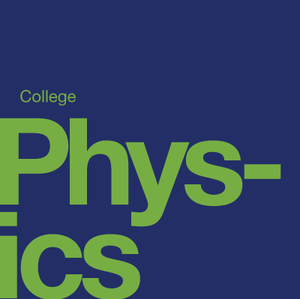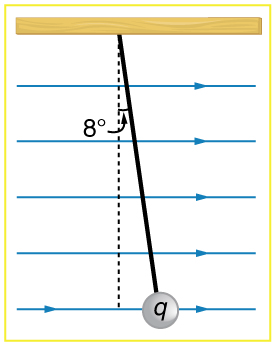# Electric Charge and Electric Field

Source: OpenStax

Student Price: FREE

This pack examines static electricity, conductors and insulators, Coulomb's Law, electric fields, electric forces in biology and more.

PE 11.11

11. As a woman walks, her entire weight is momentarily placed on one heel of her high-heeled shoes. Calculate the pressure exerted on the floor by the heel if it has an area of 1.50 $cm^2$ and the woman's mass is 55.0 kg. Express the pressure in Pa. (In the early days of commercial flight, women were not allowed to wear high-heeled shoes because aircraft floors were too thin to withstand such large pressures.) (answer in lb/in$^2$)

PE 11.11

11. As a woman walks, her entire weight is momentarily placed on one heel of her high-heeled shoes. Calculate the pressure exerted on the floor by the heel if it has an area of 1.50 $cm^2$ and the woman's mass is 55.0 kg. Express the pressure in Pa. (In the early days of commercial flight, women were not allowed to wear high-heeled shoes because aircraft floors were too thin to withstand such large pressures.) (answer in lb/in$^2$)

PE 18.2

If 1.80×10$^{20}$ electrons move through a pocket calculator during a full day's operation, how many coulombs of charge moved through it? (answer in C)

PE 18.3

To start a car engine, the car battery moves 3.75×10$^{21}$ electrons through the starter motor. How many coulombs of charge were moved? (answer in C)

PE 18.4

A certain lightning bolt moves 40.0 C of charge. How many fundamental units of charge |q$_{e}$| is this? (answer in ×10$^{20}$)

PE 18.5

Suppose a speck of dust in an electrostatic precipitator has 1.0000×10$^{12}$ protons in it and has a net charge of -5.00 nC (a very large charge for a small speck). How many electrons does it have? (answer in ×10$^{12}$)

PE 18.7

A 50.0 g ball of copper has a net charge of 2.00 µC. What fraction of the copper's electrons has been removed? (Each copper atom has 29 protons, and copper has an atomic mass of 63.5.) (answer in ×10$^{-13}$)

PE 18.8

What net charge would you place on a 100 g piece of sulfur if you put an extra electron on 1 in 10$^{12}$ of its atoms? (Sulfur has an atomic mass of 32.1.) (answer in ×10$^{-7}$)

PE 18.9

How many coulombs of positive charge are there in 4.00 kg of plutonium, given its atomic mass is 244 and that each plutonium atom has 94 protons? (answer in ×10$^{8}$ C)

PE 18.10

What is the repulsive force between two pith balls that are 8.00 cm apart and have equal charges of - 30.0 nC? (answer in ×10$^{-3}$ N)

PE 18.12

Two point charges exert a 5.00 N force on each other. What will the force become if the distance between them is increased by a factor of three? (answer in N)

PE 18.13

Two point charges are brought closer together, increasing the force between them by a factor of 25. By what factor was their separation decreased?

PE 18.14

How far apart must two point charges of 75.0 nC (typical of static electricity) be to have a force of 1.00 N between them? (answer in mm)

PE 18.15

If two equal charges each of 1 C each are separated in air by a distance of 1 km, what is the magnitude of the force acting between them? You will see that even at a distance as large as 1 km, the repulsive force is substantial because 1 C is a very significant amount of charge. (answer in ×10$^{3}$ N)

PE 18.17

Bare free charges do not remain stationary when close together. To illustrate this, calculate the acceleration of two isolated protons separated by 2.00 nm (a typical distance between gas atoms). Explicitly show how you follow the steps in the Problem-Solving Strategy for electrostatics. (answer in ×10$^{16}$ m/s$^{2}$)

PE 18.22

At what distance is the electrostatic force between two protons equal to the weight of one proton? (answer in m)

PE 18.23

A certain five cent coin contains 5.00 g of nickel. What fraction of the nickel atoms' electrons, removed and placed 1.00 m above it, would support the weight of this coin? The atomic mass of nickel is 58.7, and each nickel atom contains 28 electrons and 28 protons. (answer in ×10$^{-11}$)

PE 18.27

What is the magnitude and direction of an electric field that exerts a 2.00×10$^{-5}$ N upward force on a -1.75 µC charge? (answer in N/C downward)

PE 18.28

What is the magnitude and direction of the force exerted on a 3.50 µC charge by a 250 N/C electric field that points due east? (answer in ×10$^{-4}$ N)

PE 18.29

Calculate the magnitude of the electric field 2.00 m from a point charge of 5.00 mC (such as found on the terminal of a Van de Graaff). (answer in ×10$^{7}$ N/C)

PE 18.31

Calculate the initial (from rest) acceleration of a proton in a 5.00 ×10$^{6}$ N/C electric field (such as created by a research Van de Graaff). Explicitly show how you follow the steps in the Problem-Solving Strategy for electrostatics. (answer in ×10$^{14}$ m/sec[math]^{2}[/math)

PE 18.57

Integrated concepts Calculate the angular velocity ? of an electron orbiting a proton in the hydrogen atom, given the radius of the orbit is 0.530×10$^{-10}$ m. You may assume that the proton is stationary and the centripetal force is supplied by Coulomb attraction. (answer in ×10$^{16}$ rad/s)

PE 18.60

Integrated concepts A 5.00 g charged insulating ball hangs on a 30.0 cm long string in a uniform horizontal electric field as shown in Figure. Given the charge on the ball is 1.00 µC, find the strength of the field. Figure: A horizontal electric field causes the charged ball to hang at an angle of 8.00º. (answer in ×10$^{3}$ N/C)CC BY - OpenStax